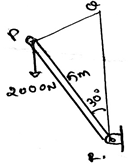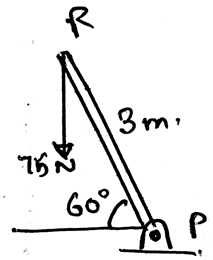# Test: Radius Of Gyration Of An Area

## 14 Questions MCQ Test Engineering Mechanics - Notes, Videos, MCQs & PPTs | Test: Radius Of Gyration Of An Area

Description
This mock test of Test: Radius Of Gyration Of An Area for Mechanical Engineering helps you for every Mechanical Engineering entrance exam. This contains 14 Multiple Choice Questions for Mechanical Engineering Test: Radius Of Gyration Of An Area (mcq) to study with solutions a complete question bank. The solved questions answers in this Test: Radius Of Gyration Of An Area quiz give you a good mix of easy questions and tough questions. Mechanical Engineering students definitely take this Test: Radius Of Gyration Of An Area exercise for a better result in the exam. You can find other Test: Radius Of Gyration Of An Area extra questions, long questions & short questions for Mechanical Engineering on EduRev as well by searching above.
QUESTION: 1

### In the calculation of the radius of gyration, we use intensity of loadings. So whenever the distributed loading acts perpendicular to an area its intensity varies __________

Solution:

The load intensity is varying linearly in the structures. Thus the intensity is not varying parabolically nor is it cubically. It cannot be a vector also. Thus the intensity is linearly varied.

QUESTION: 2

### The radius of gyration is related to moment of the body. So the calculation of the moment of the body due to the loadings involve a quantity called ____________

Solution:

The calculation of the moment of the body due to the loadings involve a quantity called moment of inertia. This is having much significance in the various fields in the engineering sector. The main types are the ‘I’ section structures which are being much used.

QUESTION: 3

### Find the moment of the force about the point R.Solution:

As we know that the moment is the cross product of the distance and the force we will try to apply the same here. We see that the perpendicular distance is 5m. Thus we get the distance. And hence multiply it with the force, 5x2000xsin30. Because the force component perpendicular to the distance need to be taken.

QUESTION: 4

How far from the shaft at P a 200N vertical force must act so as to create the same moment as produced by the 75N, at P?Solution:

As we know that the moment is the cross product of the distance and the force we will try to apply the same here. So let the distance be y, then the force of 200N will act downward. The distance perpendicular is xcos60. Thus equating the 112.5 to 200ycos60.

QUESTION: 5

Moment of Inertia is the integration of the square of the distance of the centroid and the del area along the whole area of the structure and this moment is at a distance from the rotating axis, known as radius of gyration.

Solution:

The moment of inertia of the section is the integration of the square of the distance of the centroid and the del area along the whole area of the structure. This is having much significance in the various fields in the engineering sector. The main types are the ‘I’ section structures which are being much used.

QUESTION: 6

There is perpendicular axis theorem for the calculation of radius of gyration.

Solution:

There is no perpendicular axis theorem for the radius of gyration. In spite there is the theorem as parallel axis for any area. Thus we have the theorem which is used to add the two mutually perpendicular moment of inertias.

QUESTION: 7

Radius of gyration is indirectly to the parallel axis theorem. The parallel axis theorem gives the moment of inertia ______________ to the surface of considesrance.

Solution:

Parallel axis for any area is used to add the two mutually perpendicular moment of inertias for areas. It gives a moment of inertia perpendicular to the surface of the body. That is the moment of inertia perpendicular to the surface in considerance.

QUESTION: 8

The parallel axis theorem can add any angle varied moment of inertias to give the perpendicular moment of inertia and the radius of gyration can be calculated.

Solution:

Parallel axis for any area is used to add the two mutually perpendicular moment of inertias for areas. It gives a moment of inertia perpendicular to the surface of the body. That is the moment of inertia perpendicular to the surface in considerance.

QUESTION: 9

As radius of gyration is indirectly to the parallel axis theorem, parallel axis theorem uses the ____________ of the distance.

Solution:

Parallel axis for any area is used to add the two mutually perpendicular moment of inertias for areas. It gives a moment of inertia perpendicular to the surface of the body. And uses the square of the distance from the axis of rotation.

QUESTION: 10

The distance in the parallel axis theorem is multiplied by:

Solution:

Parallel axis for any area is used to add the two mutually perpendicular moment of inertias for areas. It gives a moment of inertia perpendicular to the surface of the body. And uses the square of the distance from the axis of rotation multiplied by the area.

QUESTION: 11

One of the use of the centre of mass or centroid is as in the radius of gyration is that the net force acts at the ___________ of the loading body.

Solution:

In the moment of inertia calculations we see that the net force acts at the centroid of the loading body. That is if the loading system is in the form of the triangle then the at the distance 2 by 3 of the base the net force of the loading will act. And the load will be half the area of the loading.

QUESTION: 12

If the non-Uniform loading is of the type of parabola then for calculating the radius of gyration?

Solution:

The net force will act at the centroid of the parabola. Whether it be a parabola or the cubic curve the centroid is the only point at which the net force act. Force can’t be acted horizontally if the loading is vertical. Hence whatever be the shape of the loading, the centroid is the point of action of net force. Thus the use of centroid.

QUESTION: 13

If any external force also is applied on the structure and we are determining radius of gyration then what should we consider?

Solution:

The external forces are treated differently. They are not added by the force of the distributed loading. That is the force not only acts at the centroid always. It can be shifted also. Depending on the external forces. Thus the use of centroid or centre of mass.

QUESTION: 14

The body is sometimes acted by two or three force members and we need to find radius of gyration for the same. The difference between the two and the three force members is:

Solution:

The definition of the two force member only defines that the forces are being acted on the two points on the body. So does is the definition of the three forces members. The points of action of the three forces are three.